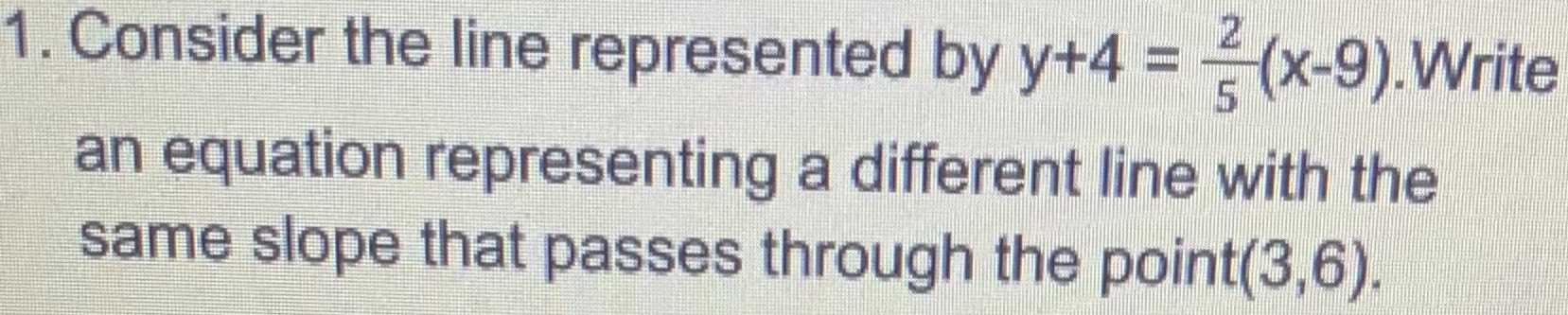### ¿Todavía tienes preguntas de matemáticas?

Pregunte a nuestros tutores expertos
Algebra
Pregunta1. Consider the line represented by $$y + 4 = \frac { 2 } { 5 } ( x - 9 )$$ . Write an equation representing a different line with the same slope that passes through the point $$( 3,6 )$$ .

y  = $$\frac{2}{5}$$x + $$\frac{24}{5}$$